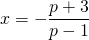# Solution assignment 10 Linear equations

### Assignment 10

For which value(s) of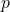does the following equation have exactly one solution: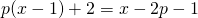### Solution

We can write this equation as: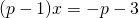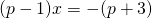This equation has only one solutionif: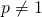This means in this case that the solution is: